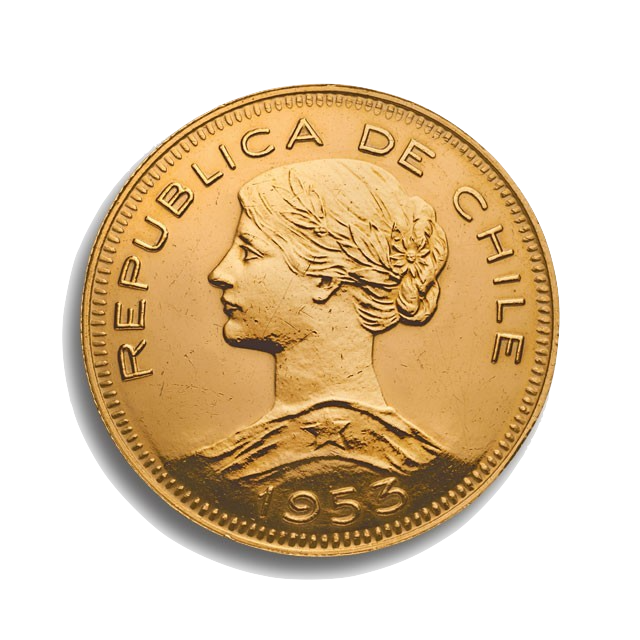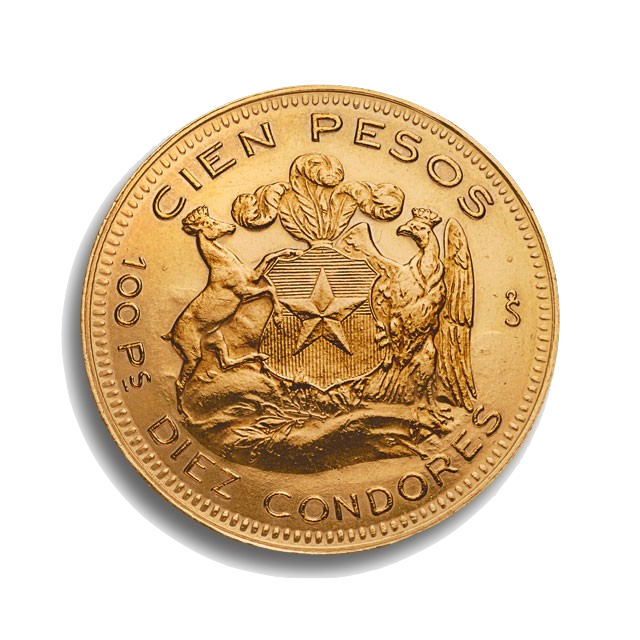April 11, 2018

## Systems are in a state

state | stāt |
noun

1. A nation or territory considered as an organized political community under one government:
• Germany, Italy, and other European states.
• United States.
2. The particular condition that someone or something is in at a specific time
• water in a liquid state.
3. The quantities of all items (circles) in a system.

## The state of the system changes with time

In any fixed time, the state is a vector

• one value for each item
• Examples:
• Concentration of H, O, Water
• Number of cats and mice
• Number of primers and DNA molecules

In R each state is a row in the data frame

The first row is the initial state

## The state today depends only on the past states

The “boxes” of the system do not change with time

The “rate” values are constant

The new state depends only on the past states, nothing else

If we know the initial state and rate constant, we can calculate everything“An intelligence knowing all the forces acting in nature at a given instant, as well as the momentary positions of all things in the universe, would be able to comprehend in one single formula the motions of the largest bodies as well as the lightest atoms in the world; to it nothing would be uncertain, the future as well as the past would be present to its eyes.”

Pierre-Simon Laplace, French mathematician and astronomer (1749 – 1827)

## These are called deterministic systems

All the future is determined
by the initial state
and the system rules

## We do experiments to discover the current state of the system

But experiments are never perfect

## Sometimes we can predict

For example we have the water formation system

## This program simulates the system

water_formation <- function(N, r1_rate=0.1,  r2_rate=0.1, H_ini=1, O_ini=1, W_ini=0) {
W <- d_W <- rep(NA, N) # Water, quantity and change on each time
H <- d_H <- rep(NA, N) # Hydrogen
O <- d_O <- rep(NA, N) # Oxygen
W <- W_ini
H <- H_ini
O <- O_ini
d_W <- d_H <- d_O <- 0 # the initial change is zero

for(i in 2:N) {
d_W[i] <-    r1_rate*H[i-1]*H[i-1]*O[i-1] - r2_rate*W[i-1]
d_O[i] <-   -r1_rate*H[i-1]*H[i-1]*O[i-1] + r2_rate*W[i-1]
d_H[i] <- -2*r1_rate*H[i-1]*H[i-1]*O[i-1] + 2*r2_rate*W[i-1]

W[i] <- W[i-1] + d_W[i]
O[i] <- O[i-1] + d_O[i]
H[i] <- H[i-1] + d_H[i]
}
return(data.frame(W, H, O))
}

## We simulate for different initial conditions

H_ini <- c(0.8, 0.9, 1.0, 1.1, 1.2)
ans <- data.frame(V1=water_formation(N=100, H_ini=H_ini)$H) par(mar=c(5,4,1,1)) plot(ans$V1, ylim=c(0.4,1.1), ylab="Hydrogen", type="b")
for(i in 2:length(H_ini)) {
ans[[i]] <- water_formation(N=100, H_ini=H_ini[i])$H points(ans[[i]], pch=i, type="b") }## The final value of H changes a little The initial values are: H_ini  0.8 0.9 1.0 1.1 1.2 and the final values after 100 steps are: ans[100,]  V1 V2 V3 V4 V5 100 0.4558742 0.5 0.543689 0.5872025 0.6307601 ## Small changes in initial state produces small changes in final state Even if we make errors, their effect is not very important ## Another system: Quadratic map • A population that reproduces and dies • Death happens in pairs (two incoming arrows) • Death rate is A/2 • Birth rate is A-1 • If we know A, we know birth and dead rate ## Formulas are easy to find d_x[i] <- (A-1) * x[i-1] - 2 * A/2 * x[i-1]*x[i-1] x[i] <- x[i-1] + d_x[i] in other words x[i] <- x[i-1] + (A-1) * x[i-1] - 2 * A/2 * x[i-1]*x[i-1] x[i] <- A * x[i-1] - A * x[i-1]*x[i-1] so finally x[i] <- A * x[i-1] * (1 - x[i-1]) ## and the program is also easy to write quad_map <- function(N, A, x_ini) { x <- rep(NA, N) x <- x_ini for(i in 2:N) { x[i] <- A * x[i-1] * (1 - x[i-1]) } return(x) } ## We simulate for different initial conditions x_ini <- c(0.4, 0.45, 0.5, 0.55, 0.6) ans <- data.frame(V1=quad_map(N=100, x_ini=x_ini, A=2)) plot(ans$V1, ylim=c(0,1), ylab="x", type="b")
for(i in 2:length(x_ini)) {
points(ans[[i]], pch=i, type="b")
}## The final state is always the sameThis is called attractor

## Behavior depends on A. Here A=3Now there are two final states.
This is a periodic attractor

## Behavior depends on A. Here A=3.5Now we have four final states.
Also a periodic attractor

## Behavior depends on A. Here A=3.8We do not see a pattern here

## Behavior depends on A. Here A=3.95Similar initial states, very different results

## Small changes in x_ini, big changes in result

Initial values:

x_ini
 0.40 0.45 0.50 0.55 0.60

Final values:

ans[100,]
            V1        V2        V3        V4        V5
100 0.07712169 0.7034133 0.8541633 0.7034133 0.4900459

## There is a Pattern

Here we see the final 500 states for different values of AHomework: do this plot. More details later

## This is called “Butterfly Effect”

The fly of a butterfly in Istanbul can produce an hurricane in Mexico

Small changes have big consequences

## But we can still say something

We cannot make exact predictions

But we can still say what is normal

What is the most probable behavior

We can identify patterns using the tools of …. (sound of drums)

## Not about games and bets

People think that probabilities are about games

Instead they are really tools for thinking

Thinking about decisions when we have incomplete information

About the meaning of our experiment results

## Do you need probabilities?

Do you travel?

• Which is the safest way to travel?
• Which is the fastest way to travel?
• How much do you want to pay for safety?
• How much do you want to pay for speed?

### Sigorta

Travel insurance, Health insurance

• how much do you pay for it?
• How much you will get paid?
• Is it worth it?

## Why do you study?

• You can work now and earn money today
• What if you fail this course?
• Why if you do not find a job?

## Will you make experiments?

• How will you understand the results?
• Are the results just “random noise”?
• What are your expected results
• Allele frequency
• Success rate of a treatment

## To understand the idea we will use games

• Cards
• Coins
• Dice (one die, many dice)

maybe other toys that are easy to understand

These are just to have easy examples

## What can happen: outcomes

Each device has a set of possible outcomes:

For example a die has the following outcomes

⚀ ⚁ ⚂ ⚃ ⚄ ⚅

## Cards

🂡 🂢 🂣 🂤 🂥 🂦 🂧 🂨 🂩 🂪 🂫 🂭 🂮 🂱 🂲 🂳 🂴 🂵 🂶 🂷 🂸 🂹 🂺 🂻 🂽 🂾 🃁 🃂 🃃 🃄 🃅 🃆 🃇 🃈 🃉 🃊 🃋 🃍 🃎 🃑 🃒 🃓 🃔 🃕 🃖 🃗 🃘 🃙 🃚 🃛 🃝 🃞 🃟

## Also Cards

♠︎♣︎♡♢

Four symbols can be used to represent DNA

A, C, G, T

## CoinsHead, Tail also written as H, T

## We know how to represent outcomes

• Coin: c("H","T")
• Dice: 1:6
• Cards/DNA: c("a","c","g","t")
• Capital Letters: LETTERS
• Small Letters: letters

## Please take a sample()

Try this

sample(LETTERS)
  "V" "P" "H" "T" "A" "W" "N" "L" "Q" "X" "M" "R" "G" "F" "D" "B" "Z" "E" "S" "K" "O" "C" "U" "Y" "I" "J"
sample(LETTERS)
  "W" "E" "S" "T" "K" "P" "Q" "U" "X" "H" "I" "V" "N" "R" "Z" "C" "B" "O" "F" "M" "Y" "L" "G" "A" "J" "D"

## sample() is shuffling

The output has the same elements of the input but in a different order

Each element appears only once

The order changes every time

## Choosing the sample size

Try this

sample(LETTERS, size=10)
  "E" "H" "V" "Y" "C" "I" "T" "M" "B" "F"

We get 10 letters. Some, but not all input elements

Each element appears only once

## Sampling many elements

Try this

sample(c("H","T"), size=10)
Error in sample.int(length(x), size, replace, prob): cannot take a sample larger than the population when 'replace = FALSE'

We run out of elements

## Sampling with replacement

Try this

sample(c("H","T"), size=10, replace=TRUE)
  "H" "T" "T" "H" "H" "H" "H" "H" "H" "H"

Each element can appear several times

Shuffle, take one, replace it on the set

Most of times we will use sample() with replace=TRUE

## Is there a pattern in the result?

table(sample(c("a","c","g","t"), size=40, replace=TRUE))
 a  c  g  t
15  6  9 10 
table(sample(c("a","c","g","t"), size=40, replace=TRUE))
 a  c  g  t
12  9 10  9 
table(sample(c("a","c","g","t"), size=40, replace=TRUE))
 a  c  g  t
6 12 11 11 

Each result is different

## Is there a pattern in the result?

table(sample(c("a","c","g","t"), size=400, replace=TRUE))
  a   c   g   t
107  92  98 103 
table(sample(c("a","c","g","t"), size=400, replace=TRUE))
  a   c   g   t
97  96 107 100 
table(sample(c("a","c","g","t"), size=400, replace=TRUE))
  a   c   g   t
94 120 103  83 

When size increases, the frequency of each letter also increases

## Is there a pattern in the result?

table(sample(c("a","c","g","t"), size=4000, replace=TRUE))
   a    c    g    t
973 1030 1025  972 
table(sample(c("a","c","g","t"), size=4000, replace=TRUE))
   a    c    g    t
988 1015 1007  990 
table(sample(c("a","c","g","t"), size=4000, replace=TRUE))
   a    c    g    t
988 1014 1002  996 

When size increases, the frequencies change less

## Is there a pattern in the result?

table(sample(c("a","c","g","t"), size=40000, replace=TRUE))
    a     c     g     t
10062  9894  9974 10070 
table(sample(c("a","c","g","t"), size=40000, replace=TRUE))
    a     c     g     t
10075  9936  9813 10176 
table(sample(c("a","c","g","t"), size=40000, replace=TRUE))
    a     c     g     t
10016  9900  9888 10196 

Each frequency is very close to 1/4 of size

## Is there a pattern in the result?

table(sample(c("a","c","g","t"), size=400000, replace=TRUE))
     a      c      g      t
99403 100022 100687  99888 
table(sample(c("a","c","g","t"), size=400000, replace=TRUE))
     a      c      g      t
100019  99766 100393  99822 
table(sample(c("a","c","g","t"), size=400000, replace=TRUE))
     a      c      g      t
100400  99938  99722  99940 

If size increases a lot, the relative frequencies are 1/4 each

## The sum of Relative Frequencies is 1

Absolute frequency is how many times we see each value
The sum of all absolute frequencies is the Total number of cases

Relative frequency is the proportion of each value in the total
The sum of all relative frequencies is always 1.

• Relative frequency = Absolute frequency/Total number of cases

## Relative Frequencies in our example

table(sample(c("a", "c", "g", "t"), size=1000000, replace=TRUE))/1000000
       a        c        g        t
0.250158 0.250116 0.250453 0.249273 

## What is the final relative frequency?

What will be each relative frequency when size is BIG

In this case we can find it by thinking

• There are 4 possible outcomes, and they are symmetrical
• There is no reason to prefer one outcome to the other
• Therefore all relative frequencies must be equal
• Therefore each one must be 1/4

This ideal relative frequency is called Probability

## Probabilities

Each device or random system may have some preferred outcomes

All outcomes are possible, but some can be probable

In general we do not know each probability

But we can estimate it using the relative frequency

That is what we will do in this course